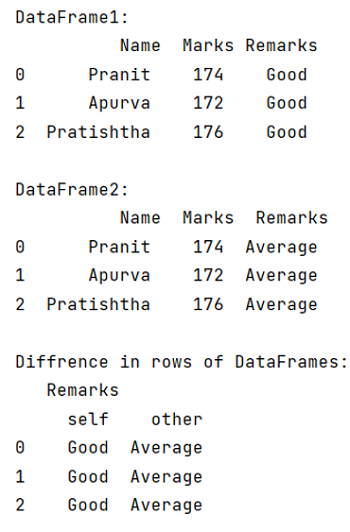# How to compare two DataFrames and output their differences side-by-side?

Given two Pandas DataFrames, we have to compare them and output their differences side-by-side. By Pranit Sharma Last updated : September 22, 2023

Pandas is a special tool which allows us to perform complex manipulations of data effectively and efficiently. Inside pandas, we mostly deal with a dataset in the form of DataFrame. DataFrames are 2-dimensional data structure in pandas. DataFrames consists of rows, columns and the data. The Data inside the DataFrame can be of any type.

Sometimes we deal with multiple DataFrames which can be almost similar with very slight changes, in that case, we might need to observe the differences between the DataFrames.

## Problem statement

Given two Pandas DataFrames, we have to compare them and output their differences side-by-side.

## Comparing two DataFrames and output their differences side-by-side

For this purpose, we will check both the DataFrames if they are equal or not. To check if the DataFrames are equal or not, we will use pandas.DataFrame.compare() method. This method is used to compare two DataFrames and to find the differences between the rows of two DataFrames. It returns the particular column where it finds the difference.

Syntax:

```DataFrame.compare(
other,
align_axis=1,
keep_shape=False,
keep_equal=False
)

# or
df1.compare(df2)
```

Here, a DataFrame will call this function and another DataFrames will be passed as parameter.

Note

To work with pandas, we need to import pandas package first, below is the syntax:

```import pandas as pd
```

Let us understand with the help of an example,

## Python program to compare two DataFrames and output their differences side-by-side

```# Importing pandas package
import pandas as pd

# Creating two dictionary
d1 = {
'Name':['Pranit','Apurva','Pratishtha'],
'Marks':[174,172,176],
'Remarks':['Good','Good','Good']
}

d2 = {
'Name':['Pranit','Apurva','Pratishtha'],
'Marks':[174,172,176],
'Remarks':['Average','Average','Average']
}

# Creating two separate DataFrames
df1 = pd.DataFrame(d1)
df2 = pd.DataFrame(d2)

# Display DataFrames
print("DataFrame1:\n",df1,"\n")
print("DataFrame2:\n",df2,"\n")

# Comparing the two DataFrames
check = df1.compare(df2)

# Display check
print("Diffrence in rows of DataFrames:\n",check)
```

### Output

The output of the above program is: